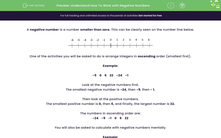# Understand How To Work with Negative Numbers

In this worksheet, students will be asked to use their knowledge of negative numbers to arrange integers and solve worded problems.Key stage:  KS 2

Curriculum topic:   Number: Number and Place Value

Curriculum subtopic:   Use Negative Numbers

Difficulty level:#### Worksheet Overview

A negative number is a number smaller than zero. This can be clearly seen on the number line below.One of the activities you will be asked to do is arrange integers in ascending order (smallest first).

Example:

-9    0    6    22    -24    -1

Look at the negative numbers first.

The smallest negative number is -24, then -9, then - 1.

Then look at the positive numbers.

The smallest positive number is 0, then 6, and finally, the largest number is 22.

The numbers in ascending order are:

-24    -9    -1    0    6    22

You will also be asked to calculate with negative numbers mentally.

Example:

The temperature on Monday morning is -4°C. The temperature on Friday morning is 1°C. How much warmer is it on Friday morning?You may be able to do this type of calculation in your head or it may help you to use a number line.